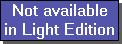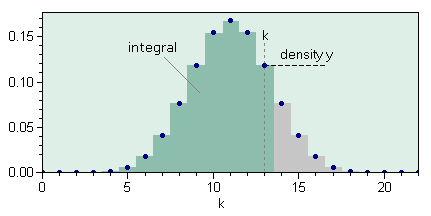The SDL Component Suite is an industry leading collection of components supporting scientific and engineering computing. Please visit the SDL Web site for more information....## BinomDistriQuantileUnit: SDL_statis Class: none Declaration: function BinomDistriQuantile (SampleSize: integer; FractionA: double; p: double; var ActualProb: double): integer;

The function BinomDistriQuantile returns the quantile of the binomial distribution for the probability p. The parameter SampleSize specifies the size of the drawn sample, the parameter FractionA controls the fraction of items marked "A" (see below). The parameter k may take any value between zero and SampleSize, the parameter FractionA is limited to the interval [0,1]. Both parameters are automatically corrected to the closest limit if an out-of-range value is specified. The variable parameter ActualProb returns the actual probability associated with the returned quantile.Background of the binomial distribution: Let's assume to have a container of a huge (infinite) amount of balls with a certain fraction (parameter FractionA) of them being marked by "A", the others being marked by "B". The binomial distribution provides an answer to the question "what is the probability to get k "A" balls when drawing SampleSize balls from the container?". The function BinomDistriQuantile calculates the maximum number of "A" balls which can be expected with a probability of p.

Last Update: 2020-Aug-04